# Number of Longest Increasing Subsequences | (DP-47)

Problem Statement:

Number of Longest Increasing Subsequences

Given an array, ‘Arr’ of length ‘n’, count the number of longest increasing subsequences (LIS).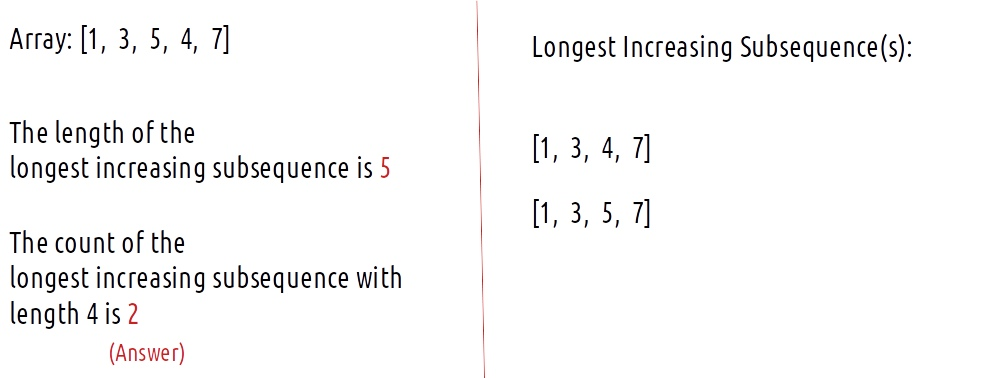Solution

Intuition:

Now let us revise the approach for finding the LIS as discussed in Printing Longest Increasing subsequence. Readers are highly advised to understand that approach first. In that solution, we write two nested loops to get a dp array whose maximum element gives us the length of the LIS as shown below: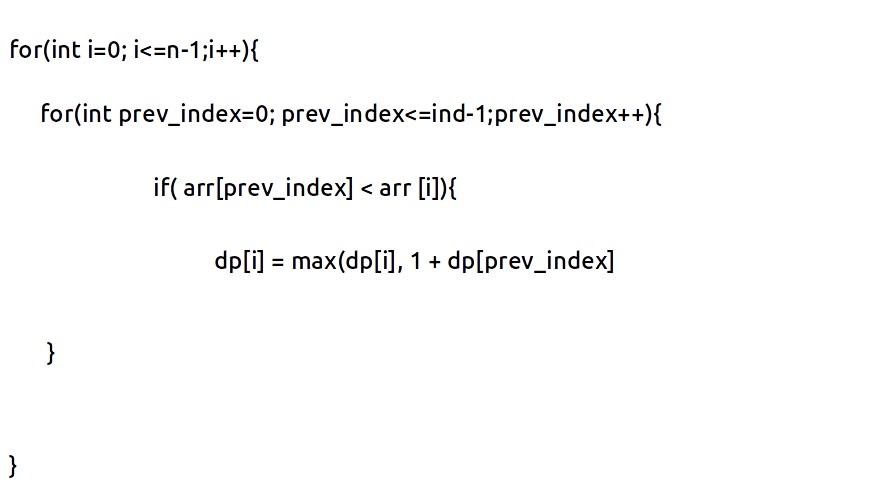Now, what does this dp[i] represent? It represents the LIS in the array ‘arr’ from index 0 to index i in the array ending with element arr[i]. Therefore the maximum value of dp[i] in gives us the LIS of the array.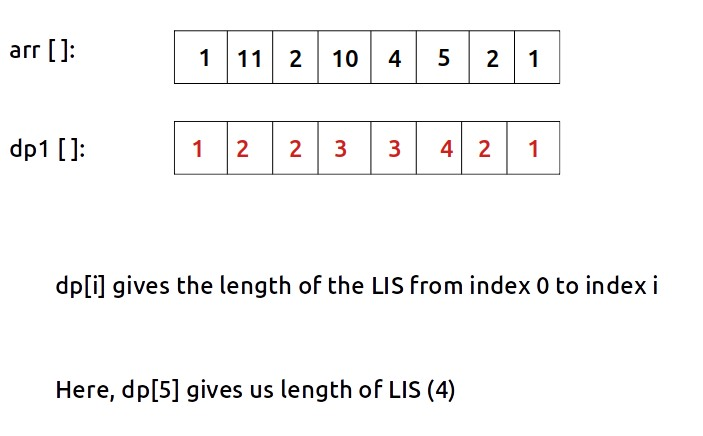Now, after understanding the approach to finding the LIS, let us revisit the problem of counting the number of longest increasing subsequences of the array.

Let us take a new array ct[ ] to calculate the count and initialize it to 1. Then ct[i] will be the count of the number of longest increasing subsequences where each LIS ends where arr[i] is the last element of the subsequence.

Let us now understand the approach to get the values of the ct[ ] array, i.e to count the number of LIS. For that we will consider the below example:

Logic and approach for the dp and ct array[ ]

Initial example:

The initial example is given below and the dp[ ] and ct[ ] array are initialized to 1.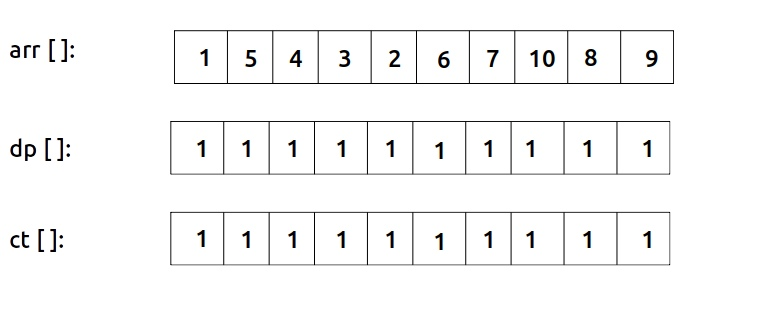Now, we will iterate over the array and one by one see the final value of the dp[ ] and ct[ ] array. At this stage, it is super important to have a crystal clear understanding of what dp[i] and ct[i] means. Therefore their definitions are given in the below figure: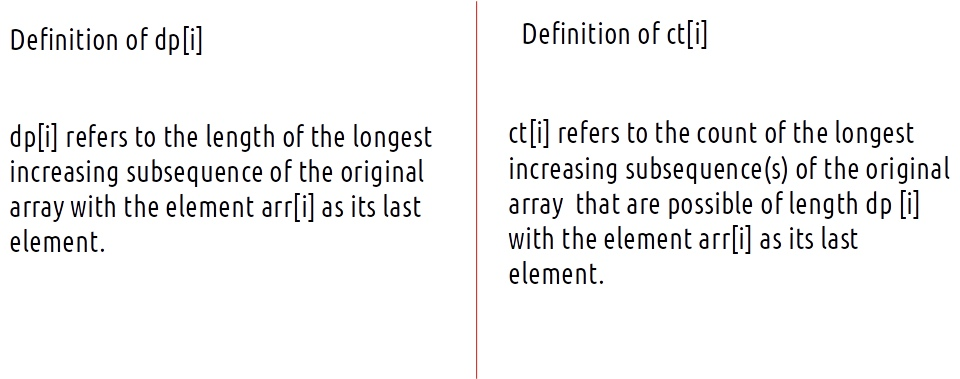• For i = 0,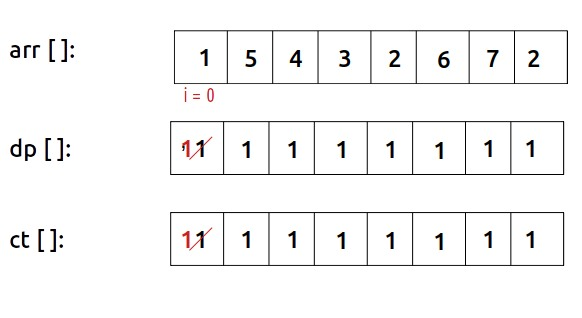We know that dp[i] and ct[i] will be 1 as they will be the only member of the unique LIS.

• For i=1,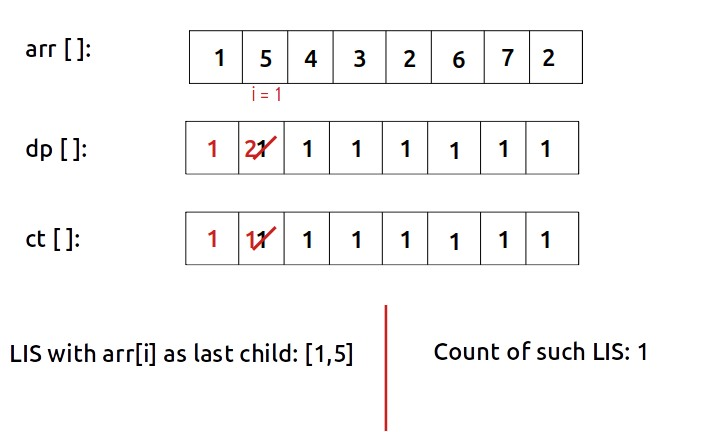We know that 5 is greater than 1, so we can append it, therefore dp will be 2 and the count still remains 1.

Similar is the case for i = 2,3 and 4.• For i=5,

This is an interesting case and we will break it into pieces. We are setting a nested loop, where i =5 and prev_index(say j) runs from 0 to 4.

• Initially j=0,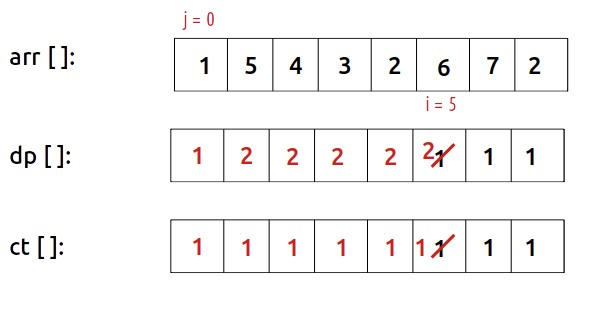At j=0, arr[j]<arr[i] and dp[j+1]>dp[i], therefore we can place 1 before 6 in the subsequence([1, 6]) to get LIS of length 2 in such a case, ct[i] will be ct[j]. In other words, the number of ways of getting LIS ending at arr[i], i.e ct[i] is equal to the number of ways of getting ct[j]. (ct[i] = ct[j]).

At j=1, a similar case will follow, as arr[j]<arr[i] and dp[j+1]>dp[i], therefore we again update ct[i] to the value of ct[j].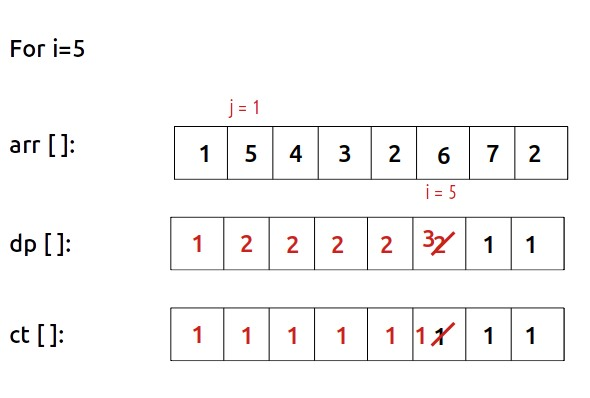Now let us see what happens at j =2,

At j=2, arr[j]<arr[i], therefore we can consider placing before 6 but the length o0f LIS by placing the 4 before 6 will be 3, which is the existing value of dp[i]. In such a case we need to consider both the existing and the new LIS. Therefore, we will update ct[i] = ct[i] + ct[j], i.e 2 to count both the LIS ( [1, 5, 6] and [1, 4, 6]).Similar will be the case for j=3, and j=4 as both will give us LIS of length 3 so we will count them as well.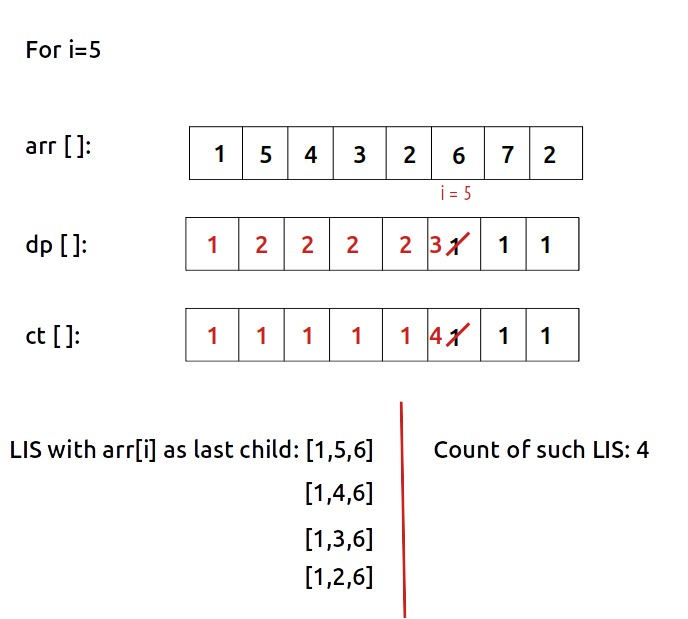• For i=6,

When j=5, arr[j]<arr[i], so we can add 6 before 7 for LIS, so we can again have 4 LIS.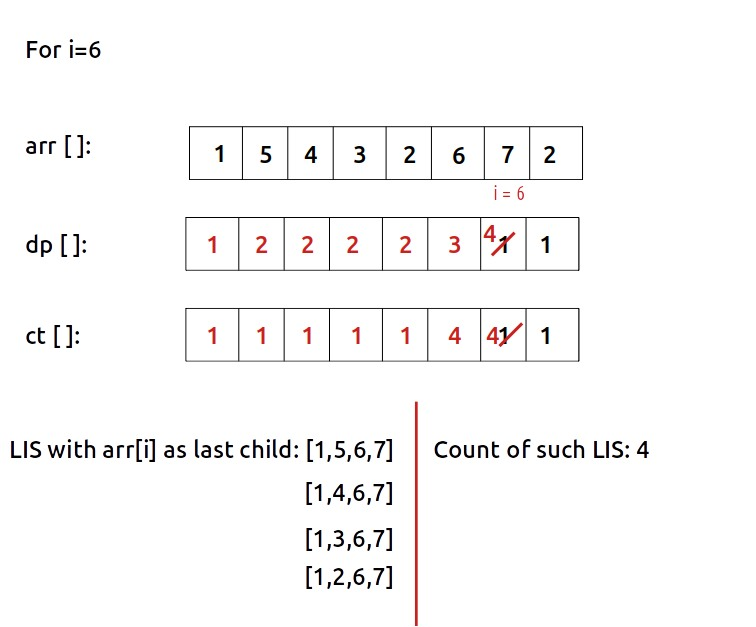Approach:

If we closely see the example we see two patterns for the nested loop conditions. We will always consider element at prev_index( say j) to place before element at index i only if arr[j] < arr[i]. Now, there arises two conditions:

1. if( arr[j] < arr[i] && dp[j+1] > dp[i]), in this case we get a new LIS of greater length, therefore the number of LIS ending at arr[i], is the same as number of LIS ending at arr[j] as we simply append the element arr[j] to all those LIS. In simple terms, ct[i] = ct[j]. Try to dry run this example to understand: [1, 2, 3],
2. if( arr[j] < arr[i] && dp[j+1]==dp[i]) in this case we get a new LIS of same length at which the ct[i] is originally holding the value for. Therefore the new ct[j] value will be the number of LIS that was given by its original value plus the number of LIS that ends at element arr[j] at length dp[j]. In simple terms, ct[i] = ct[i] + ct[j]. Try to dry run this example to understand: [1, 5, 6, 10].

Based on these two conditions we can easily calculate the ct[ ]n array and return the ct[ ] value for the maximum value(s) of the dp[ ] array.

Code:

## C++ Code

``````#include <bits/stdc++.h>
using namespace std;

int findNumberOfLIS(vector<int>& arr){

int n = arr.size();

vector<int> dp(n,1);
vector<int> ct(n,1);

int maxi = 1;

for(int i=0; i<=n-1; i++){
for(int prev_index = 0; prev_index <=i-1; prev_index ++){

if(arr[prev_index]<arr[i] && dp[prev_index]+1>dp[i]){
dp[i] = dp[prev_index]+1;
//inherit
ct[i] = ct[prev_index];
}
else if(arr[prev_index]<arr[i] && dp[prev_index]+1==dp[i]){
//increase the count
ct[i] = ct[i] + ct[prev_index];
}
}
maxi = max(maxi,dp[i]);
}

int nos =0;

for(int i=0; i<=n-1; i++){
if(dp[i]==maxi) nos+=ct[i];
}

return nos;
}

int main() {

vector<int> arr = {1,5,4,3,2,6,7,2};

cout<<"The count of LIS is "
<<findNumberOfLIS(arr);

return 0;
}
``````

Output:

The count of LIS is 4

Time Complexity: O(N*N)

Reason: There are two nested loops that are run twice.

Space Complexity: O(N)

Reason: We are only using two rows of size n.

## Java Code

``````import java.util.*;

class TUF{
static int findNumberOfLIS(int[] arr){

int n = arr.length;

int[] dp= new int[n];
int[] ct= new int[n];

Arrays.fill(dp,1);
Arrays.fill(ct,1);

int maxi = 1;

for(int i=0; i<=n-1; i++){
for(int prev_index = 0; prev_index <=i-1; prev_index ++){

if(arr[prev_index]<arr[i] && dp[prev_index]+1>dp[i]){
dp[i] = dp[prev_index]+1;
//inherit
ct[i] = ct[prev_index];
}
else if(arr[prev_index]<arr[i] && dp[prev_index]+1==dp[i]){
//increase the count
ct[i] = ct[i] + ct[prev_index];
}
}
maxi = Math.max(maxi,dp[i]);
}

int nos =0;

for(int i=0; i<=n-1; i++){
if(dp[i]==maxi) nos+=ct[i];
}

return nos;
}

public static void main(String args[]) {

int[] arr = {1,5,4,3,2,6,7,2};

System.out.println("The count of LIS is "+findNumberOfLIS(arr));

}
}
``````

Output:

The count of LIS is 4

Time Complexity: O(N*N)

Reason: There are two nested loops that are run twice.

Space Complexity: O(N)

Reason: We are only using two rows of size n.

Special thanks to Anshuman Sharma for contributing to this article on takeUforward. If you also wish to share your knowledge with the takeUforward fam, please check out this articleIf you want to suggest any improvement/correction in this article please mail us at [email protected]